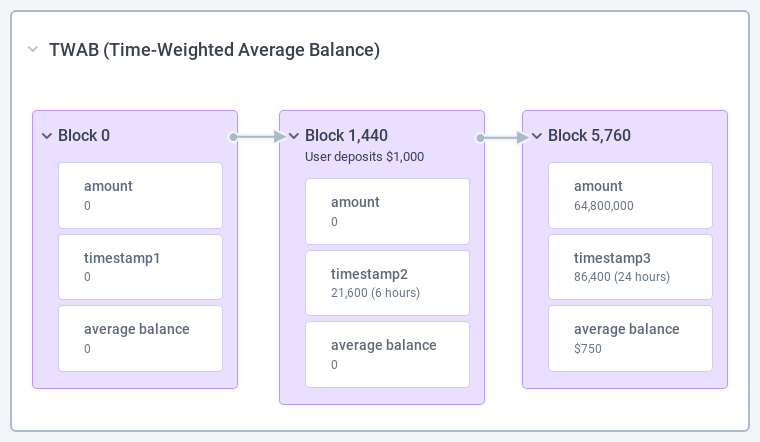# TWAB Rewards

After launching PoolTogether V4 in October 2021 we knew that prizes alone would not be enough to attract deposits and keep growing the TVL (Total Value Locked) in the protocol. Only two months after the launch, the TVL on Polygon was around \$23 Million. However, deposits were not growing substantially above this level. We needed another way to reward our users and keep the TVL growing.

### Challenge

Build a smart contract that would allow anyone to distribute rewards in the form of a ERC20 token to prize pool depositors.

### Solution

I built a smart contract that leverages the TWAB (Time Weighted Average Balance) introduced in V4. This mechanism allows us to reward users on a per epoch basis. The longer they hold their deposits, the more rewards they will receive. Rewards are claimable at the end of each epoch and users do not have to stake their deposits to be eligible to claim these rewards.

## Technical Overview

TWAB, or Time-Weighted Average Balance, is a concept wholly inspired by Uniswap’s Time-Weighted Average Price. We use TWAB to calculate the average deposit held by a user during a specific time period.

Here’s how it works.

Users deposit ERC20 tokens, usually a stablecoin like USDC, into a prize pool and then receive tokens that represent their deposit. When the tokens are minted a new TWAB is recorded.

A TWAB record is a tuple made with an amount and a timestamp. The amount stores the cumulative Time-Weighted Balance. This value is always increasing. The timestamp is taken from the block in which the transaction is mined and the TWAB recorded. The TWAB is recorded before a user balance changes. Which gives us the following formula:

``````  new TWAB amount = last TWAB amount + current balance * (current time - last TWAB timestamp)
new TWAB timestamp = current block timestamp
``````

Let’s review a concrete example:For the sake of simplicity, we will base our example on a 24 hour timeline, also called epoch, that starts at block 0 and ends at block 5,760 since a new block is mined every 15 seconds.

Our user decides to deposit at block 1,440, or 6 hours into our timeframe. At this time, the recorded amount is still 0. Remember, the TWAB amount is recorded before a user balance changes and the current block timestamp is 21,600.

We then retrieve the user balance at the end of the 24 hour timeframe, block 5,760. Using the formula above, the TWAB amount is:

``````  TWAB amount = 0 + 1000 * (86400 - 21600) = 64800000
``````

With this new recorded TWAB amount we can calculate the user average balance during this 24 hour timeframe with the following formula:

``````  average balance =
(last TWAB amount - first TWAB amount) / (last TWAB timestamp - first TWAB timestamp)
``````

Which gives us:

``````  average balance = (64800000 - 0) / (86400 - 0) = 750
``````

### How to calculate rewards

Now that we have reviewed how to compute a TWAB, we can easily calculate the rewards for our user.

Let’s take our previous example with a reward pot of \$1,000 and two users that deposit \$1,000 into the pool at different timestamps.The following formula is used to compute how many rewards a user is entitled to receive:

``````  reward amount = (reward pot * user average balance) / average total supply;
``````

At block 5,760 Bob has an average balance of \$750 and Alice has \$250. Since they are the only two users who deposited, the average total supply during the 24 hour period is \$1000.

Knowing this, we can easily calculate the amount of rewards per user:

``````  bob rewards = (1000 * 750) / 1000 = 750
alice rewards = (1000 * 250) / 1000 = 250
``````

### But sir, what about gas cost?

As you may have guessed by now, the TWAB calculation and recording incurs an overhead to Ticket mint, transfer and burn costs. Assuming a cold account is an address that has not held any tokens, and a hot account is one that has held tokens in the past, we have some approximate gas usage:

• mint (cold): 140k
• transfer (hot -> cold): 120k
• transfer (hot -> hot): 90k

After testing transactions on Rinkeby, we observe the following results:

So we can safely assume that the base cost to claim an epoch is 94k gas and 16k gas for each additional epoch claimed.

### Skills

LanguagesSolidity

Smart Contracts ToolingHardhat